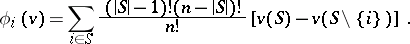# Shapley value

(diff) ← Older revision | Latest revision (diff) | Newer revision → (diff)
A vector function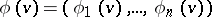defined on the set of characteristic functions of-person games and satisfying the following axioms: 1) (efficiency) if a coalitionis such that for any coalitionthe equality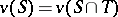holds, then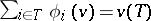; 2) (symmetry) ifis a permutation of the set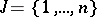and if for any coalitionthe equalityholds, then; and 3) (linearity)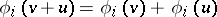. These axioms were introduced by L.S. Shapley  for an axiomatic definition of the expected pay-off in a cooperative game. It has been shown that the only vector function satisfying the axioms 1)–3) is